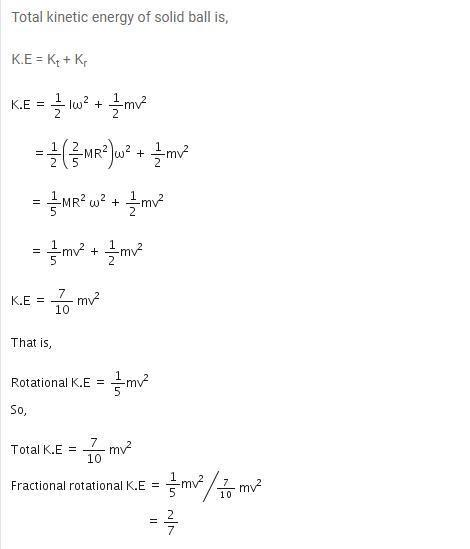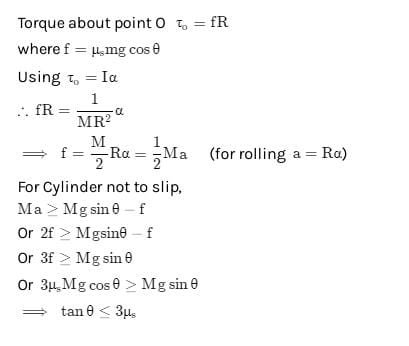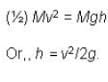NEET  >  Test: Rolling Motion

# Test: Rolling Motion

Test Description

## 10 Questions MCQ Test Physics Class 11 | Test: Rolling Motion

Test: Rolling Motion for NEET 2022 is part of Physics Class 11 preparation. The Test: Rolling Motion questions and answers have been prepared according to the NEET exam syllabus.The Test: Rolling Motion MCQs are made for NEET 2022 Exam. Find important definitions, questions, notes, meanings, examples, exercises, MCQs and online tests for Test: Rolling Motion below.
Solutions of Test: Rolling Motion questions in English are available as part of our Physics Class 11 for NEET & Test: Rolling Motion solutions in Hindi for Physics Class 11 course. Download more important topics, notes, lectures and mock test series for NEET Exam by signing up for free. Attempt Test: Rolling Motion | 10 questions in 10 minutes | Mock test for NEET preparation | Free important questions MCQ to study Physics Class 11 for NEET Exam | Download free PDF with solutions
 1 Crore+ students have signed up on EduRev. Have you?
Test: Rolling Motion - Question 1

### A spherical solid ball rolls on a table. What fraction of its total K.E. is rotational K.E. ?

Detailed Solution for Test: Rolling Motion - Question 1Test: Rolling Motion - Question 2

### A body is rolling down an inclined plane without slipping. How does the acceleration of the rolling body depend on its radius?

Detailed Solution for Test: Rolling Motion - Question 2

The acceleration of a rolling body is independent of its mass and radius.

Test: Rolling Motion - Question 3

### Acceleration (a) of a body of radius R rolling down an inclined plane of inclination is given by

Test: Rolling Motion - Question 4

What is the moment of inertia of a solid sphere about its diameter?

Detailed Solution for Test: Rolling Motion - Question 4

We have to consider solid sphere contribution of infinite small solid cylinder.
Icylinder = ½ MR²
dI = ½ dmr²
dm = pdV
dV = πr²dx
By substituting dV into dm
dm = pπr²dx
Now dm into dI
dI = ½ r²pπr²dx = ½ pπr⁴dx
Now, r² = R²-x²
dI = ½ pπ(R²-x²)²dx
Integrate both sides
I = ½ pπ 16/15 R²
P = M/V
P = M/4/3πR³ (density of sphere)
After substituting
I = 2/5MR2

Test: Rolling Motion - Question 5

What is the coefficient of friction when a cylinder rolls down without slipping on an inclined plane?

Detailed Solution for Test: Rolling Motion - Question 5Test: Rolling Motion - Question 6

If a solid cylinder rolls up along a inclined plane with an initial velocity v. It will rise up to a height h equal to

Test: Rolling Motion - Question 7

What will be the speed of center of mass, if a solid sphere of radius 20 cm which rotates at 2 rps along a straight line?

Detailed Solution for Test: Rolling Motion - Question 7

V = rw
V = 20 × 10-2 × 2π(2)
V = 2.51 m/s

Test: Rolling Motion - Question 8

Two discs A and B are mounted on a vertical axle. Suppose the discs have moment of inertia as I and 2 I respectively about an axis. Disc A is given angular velocity ω, using the potential energy of a spring compressed by x1. Similarly the potential energy of a spring compressed by x2 gives an angular velocity of 2ω to Disc B. Considering that both the discs rotate in same direction .Find the ratio x1/ x2 :

Test: Rolling Motion - Question 9

A small cylinder rolling with a velocity v along a horizontal surface encounters a smooth inclined surface. The height ‘h’ up to which the cylinder will ascend is

Detailed Solution for Test: Rolling Motion - Question 9

A body can roll along a surface only if the surface is rough. The body will roll up to the foot of the inclined smooth surface. It will continue to spin with the angular speed it has acquired, and will slide up to a certain height, maintaining its spin motion throughout the smooth surface. Its translational kinetic energy alone is responsible for its upward motion along the smooth incline so that the height up to which it will rise is given byTest: Rolling Motion - Question 10

The kinetic energy of a rolling wheel is

Detailed Solution for Test: Rolling Motion - Question 10

The total KE is the sum of translational KE and rotational KE.

## Physics Class 11

126 videos|449 docs|210 tests
 Use Code STAYHOME200 and get INR 200 additional OFF Use Coupon Code
Information about Test: Rolling Motion Page
In this test you can find the Exam questions for Test: Rolling Motion solved & explained in the simplest way possible. Besides giving Questions and answers for Test: Rolling Motion, EduRev gives you an ample number of Online tests for practice

## Physics Class 11

126 videos|449 docs|210 tests

### How to Prepare for NEET

Read our guide to prepare for NEET which is created by Toppers & the best Teachers